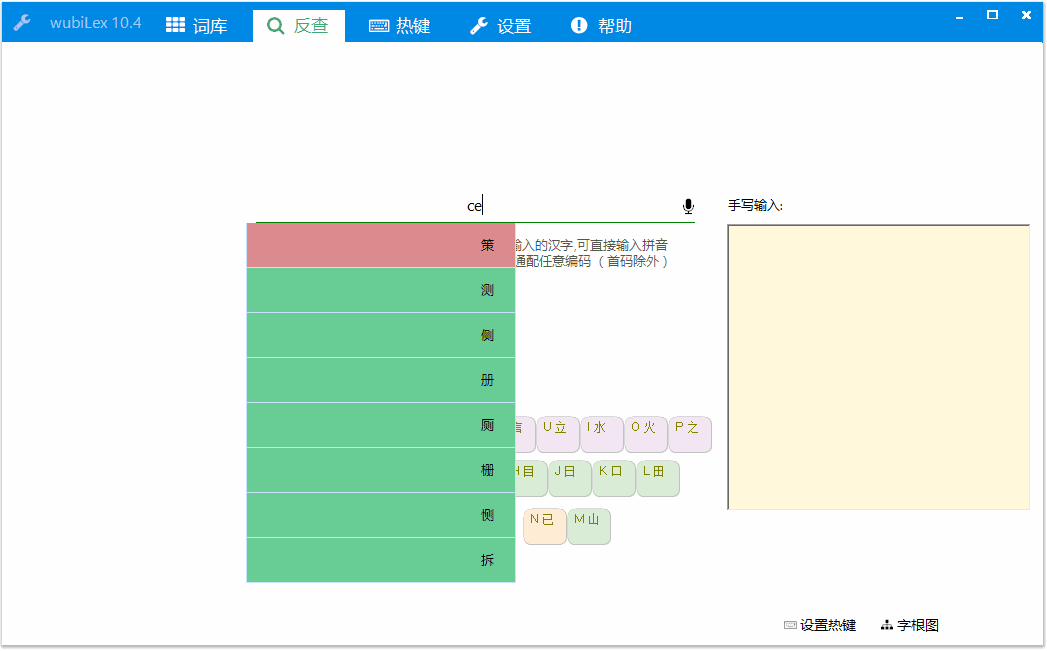# aardio + AutoHotkey 混合编程，制作漂亮界面

aardio 小、轻、快，专注于 Windows 桌面软件快速开发。在 aardio 中可以调用很多编程语言，例如 C语言、C 、C#、Java、Python、R、Javascript、Node.Js、Fortran、VB、Flash ActionScript、PHP、VBScript、PowerShell、NewLISP、AutoLISP、Delphi、FreeBASIC、Ruby、Rust、Julia、Nim、Go 语言、批处理 ...... 请参考《 aardio 调用第三方编程语言示例》1、双击打开：aardio 工具 / 扩展库
2、扩展库名字输入 ahk，搜索结果中双击 ahk 扩展库 —— 打开最新范例。aardio 提供了 ahk 扩展库可用于嵌入 AutoHotkey 并与 AutoHotkey 交互调用，支持 AutoHotkey v1 以及 AutoHotkey v2 版本。

``import win.ui;/*DSG{{*/var winform = win.form(text="嵌入 AutoHotkey v1 演示")winform.add(edit={cls="edit";left=17;top=18;right=741;bottom=445;edge=1;multiline=1;z=1})/*}}*///导入 AutoHotkey v1 扩展库import ahk; /*导出允许AutoHotkey 调用的 aardio 函数（函数名必须以 on 开头）。*/ahk.onTest = function(param){    winform.edit.print( "winform.onTest被ahk调用",param );     winform.show();}//运行ahk代码ahk.run( `#Persistent ;保持 AHK 线程不退出#NoTrayIcon ;不显示托盘图标#include <aardio>  ;启动 AHK 代码中必须加入这句才能与 aardio 交互; 定义函数与 aardio 有点像，aardio 一般会写为 GetPoint = function(hwnd){ return value;}GetPoint(){        ; 直接调用aardio函数，支持任意类型、不定个数的调用参数    aardio.onTest({ x : 2, y : 3 })    return { x : 2, y : 3 }}`);/*直接调用ahk里定义的全局函数，参数和返回值自动支持各种数据类型，就像调用普通aardio函数。是不是很神奇？！*/var point = ahk.GetPoint();winform.edit.print("调用ahk函数的返回值：",point);win.loopMessage(); ``

``import ahk; import dotNet.desktop;import win.ui;/*DSG{{*/var winform = win.form(text="虚拟桌面切换";right=599;bottom=399)winform.add(edit={cls="edit";left=17;top=18;right=565;bottom=374;edge=1;multiline=1;z=1})/*}}*///导出 AutoHotkey 函数ahk.onSwitch = function(index){ winform.edit.print("已切换桌面到：",index);//虚拟桌面在键盘钩子中需要通过返回异步函数异步调用。return function() {dotNet.desktop.get(index).MakeVisible()}} //运行 AutoHotkey 代码注册热键ahk.run( `#Persistent#NoTrayIcon #include <aardio> ^j:: aardio.onSwitch(1)^k:: aardio.onSwitch(2)`);winform.show();win.loopMessage();``

``ahk.import("path/libName"); ``

``\AutoHotkey\Lib\path\libName.ahk ``

``%A_MyDocuments%\AutoHotkey\Lib\path\libName.ahk ``

``#include <path/libName> ``

``//直接修改 AHK 全局变量的值ahk.setGlobal("gVar","测试一下")``

``//运行 AHK 代码ahk.run( `#Persistent ;#NoTrayIcon ;#include <aardio>  ;global gVar := "<?="允许嵌入 aardio 代码"?>"  ; `);//直接修改 AHK 全局变量的值ahk.setGlobal("gVar","测试一下")``

``import win.ui;/*DSG{{*/var winform = win.form(text="嵌入 AutoHotkey v2 演示")winform.add(edit={cls="edit";left=8;top=10;right=749;bottom=452;edge=1;multiline=1;z=1})/*}}*/winform.show();import ahk.thread;var ahk2 = ahk.thread();//直接定义允许 AHK 调用的 aardio 函数（函数名以on开头）ahk2.onTest = function(param){    winform.edit.print( "winform.onTest被ahk调用",param );     return 123}//运行 AHK 代码ahk2.run( `#NoTrayIcon ;不显示托盘图标Persistent( true)  ;保持ahk线程不退出 ; 定义函数与 aardio 有点像，aardio 一般会写为 GetPoint = function(hwnd){ return value;}GetPoint(){        ; 直接调用aardio函数，支持任意类型、不定个数的调用参数    aardio.onTest({ x : 2, y : 3 })    return { x : 2, y : 3 }}`);/*直接调用ahk里定义的全局函数，参数和返回值自动支持各种数据类型，就像调用普通aardio函数。是不是很神奇？！*/var point = ahk2.GetPoint();winform.edit.print("调用ahk函数的返回值：",point);win.loopMessage();  ``

``import win.ui;/*DSG{{*/var winform = win.form(text="嵌入 AutoHotkey v1")winform.add(edit={cls="edit";left=17;top=18;right=734;bottom=444;edge=1;multiline=1;z=1})/*}}*/import ahk; ahk.onTest = function(param){    winform.edit.print( "winform.onTest被ahk调用",param );     winform.show();}ahk.run( `#Persistent#NoTrayIcon#include <aardio>GetPoint(){           ; 命令式语法 = 号后面是字符串，变量要放到百分号里    POINT_TYPE =      (        UInt x;        UInt y;    ) ; 首尾两个括号都必须独占一行，用于包含多行字符串             ; 新语法 := 后面是表达式,和其他编程语言类似字符串放引号里,变量不用放百分号里    point := Struct( POINT_TYPE ) ;         ; 取结构体指针，aardio 里调用 API 时结构体总是自动转为指针，不需要写这些代码    ptr := point[]       ; DllCall("user32.dll\GetCursorPos", "Ptr", ptr)       GetCursorPos(ptr) ;     ; 直接调用aardio函数，支持任意类型、不定个数的调用参数    aardio.onTest({ x : point.x, y : point.y }); 命令式语法, 百分号加一个空格表示后面是表达式MsgBox, % "x:" point.x ",y:" point.y    return { x : point.x, y : point.y }}`); /*直接调用ahk里定义的全局函数*/var point = ahk.GetPoint();winform.edit.print("调用ahk函数的返回值：",point);win.loopMessage(); ``

``// 当然上面的代码用 aardio 直接写会更简单，例如：var point = {int x;int y};//不用声明直接调用 API，不用写参数类型这些::User32.GetCursorPos(point);``

aardio 可以免声明直接调用 WinAPI ，不需要指定参数类型。

``import fonts.fontAwesome;import win.ui;/*DSG{{*/var winform = win.form(text="嵌入autohotkey演示";right=757;bottom=467)winform.add(button={cls="plus";text="暂停AHK";left=19;top=11;right=109;bottom=41;bgcolor=-6960024;font=LOGFONT(name='FontAwesome';charset=0);z=2};edit={cls="edit";left=17;top=56;right=729;bottom=443;edge=1;multiline=1;z=1})/*}}*/import ahk;var handle = ahk.run( `#Persistent ;保持ahk线程不退出#NoTrayIcon ;不显示托盘图标#include <aardio> ;启动ahk代码中必须加入这句才能与aardio交互::hj::run无乱码`);winform.button.onMouseClick = function(){if(winform.button.text=="暂停AHK"){thread.suspend(handle)winform.button.text = "继续AHK"}else {thread.resume(handle)winform.button.text = "暂停AHK"}}winform.button.skin({color={active=0xFFFFFFFF;default=0xFF000000;hover=0xFFFFFFFF};});winform.show();win.loopMessage();``

aardio 制作图形界面很方便，请参考 aardio 开始页的教程《使用 plus 控件制作精美界面》。下面我们看一下 aardio 制作的一些开源软件。